# CEC

The R Package CEC performs clustering based on the cross–entropy clustering (CEC) method, which has been recently developed with the use of information theory. The main advantage of CEC is that it combines the speed and simplicity of k-means with the ability to use various Gaussian mixture models and reduce unnecessary clusters.

The CEC package is a part of CRAN repository and it can be installed by the following command:

``````install.packages("CEC")
library("CEC")
``````

The basic usage comes down to the function `cec` with two arguments: input data (`x`) and the initial number of centers (`centers`):

``````cec(x = ..., centers = ...)
``````

Below, a simple session with R is presented, where the component (waiting) of the Old Faithful data set is split into two clusters:

``````library("CEC")
attach(faithful)
cec <- cec(matrix(faithful\$waiting), 2)
print(cec)
``````

As the main result, CEC returns data cluster membership `cec\$cluster`. The following parameters of clusters can be obtained as well:

• means (`cec\$centers`)
• covariances (`cec\$covariances.model`)
• probabilities (`cec\$probability`)

Additional information concerning the number of iterations, cost (energy) function and the number of clusters at each iteration are also available.

Below, a session of R is presented which shows how to use the above parameters for plotting the data and the Gaussian models corresponding to the clusters.

``````hist(faithful\$waiting, prob = T, main = "Time between Old Faithful eruptions", xlab = "Minutes",
col = "lightgray", border = 0, ylim = c(0, 0.05))

for(i in c(1:2))
curve(cec\$probability[i] * dnorm(x, mean = cec\$centers[i], sd = sqrt(cec\$covariances.model[[i]])),
add = T, col = i + 1, lwd = 2)
``````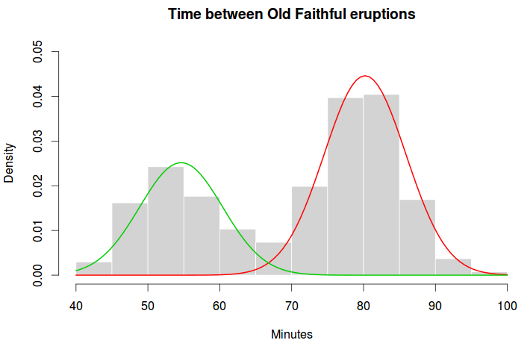The CEC method, analogously to k-means, depends on the initial clusters memberships. Therefore, the initialization should be started a few times, which can be achieved using the `nstart` parameter.

``````cec <- cec(x = ...,  centers = ..., nstart = ...)
``````

Multiple threads can be used to speed up clustering (when `nstart > 1` ). It's driven by the `threads` parameter (more details in the package manual).

``````cec <- cec(..., nstart = 100, threads = 4)
``````

The initial locations of the centers can be chosen either randomly or using the k-means++ method and it's driven by the `centers.init` parameter which can take one of the two values: `"random"` or `"kmeans++"`.

Two essential parameter, in the context of CEC method, are `card.min` and `iter.max` that express minimal cluster size - the number of points, below which, the cluster is removed and the maximum number of iterations at each start, respectively.

One of the most important properties of the CEC algorithm is that it can be applied to various Gaussian models. The CEC package includes the implementation of six Gaussian models, which can be specified by the parameter `type`.

## Implemented Gaussian models (families)

### General Gaussian distributions

`type = "all"`

The general Gaussian CEC algorithm gives similar results to those obtained by the Gaussian Mixture Models. However, the CEC does not use the EM (Expectation Maximization) approach for minimization but a simple iteration process (Hartigan method). Consequently, larger data sets can be processed in shorter time.

The clustering will have a tendency to divide the data into clusters in the shape of ellipses (ellipsoids in higher dimensions).

``````data("fourGaussians")

cec <- cec(fourGaussians, centers = 10, type = "all", nstart = 20)

plot(cec, asp = 1)
``````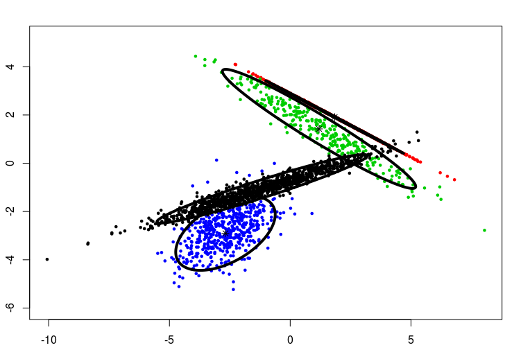### Spherical CEC

`type = "spherical"`

The original distribution will be estimated by spherical (radial) densities, which will result in splitting the data into circle-like clusters of arbitrary sizes (balls in higher dimensions).

``````data("Tset")

cec <- cec(x = Tset, centers = 10, type = "spherical", nstart = 5)

plot(cec, asp = 1)
``````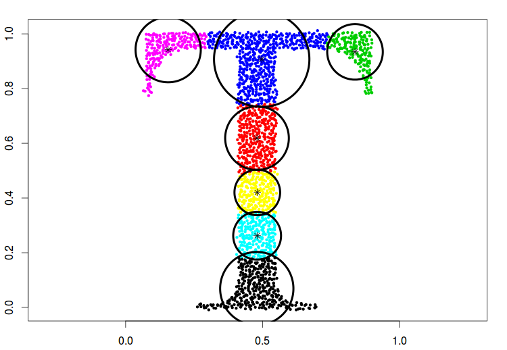### Spherical CEC with fixed radius

`type = "fixedr"`

Similarly to the general spherical model, the dataset will be divided into clusters resembling full circles, but with the radius determined by the `param` argument.

``````data("Tset")

cec <- cec(x = Tset, centers = 10, type = "fixedr", param = 0.01, nstart = 20)

plot(cec, asp = 1)
``````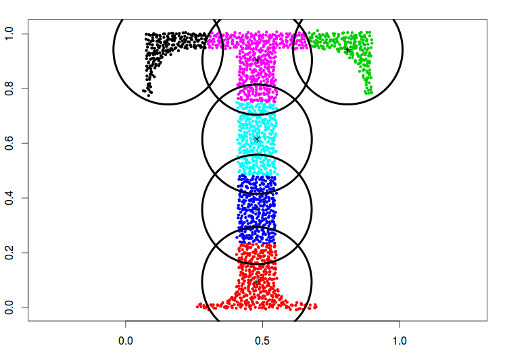### Diagonal CEC

`type = "diagonal"`

In this case, the data will be described by ellipses for which the main semi-major axes are parallel to the axes of the coordinate system.

``````data("Tset")

cec <- cec(x = Tset, centers = 10, type = "diagonal", nstart = 5)

plot(cec, asp = 1)
``````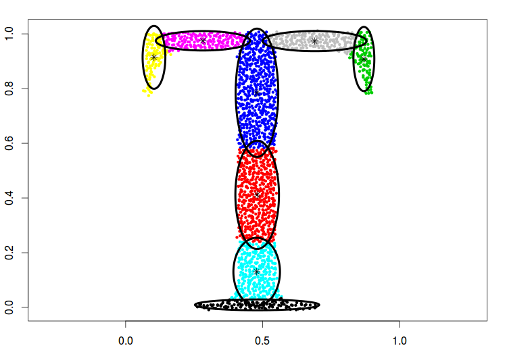### Fixed covariance CEC

`type = "covariance"`

This model contains Gaussians with fixed covariance matrix.

``````data("Tset")

cec <- cec(x = Tset, centers = 10, card.min = '10%', type = "covariance",
param = matrix(c(0.04, 0, 0, 0.01), 2))

plot(cec, asp = 1)
``````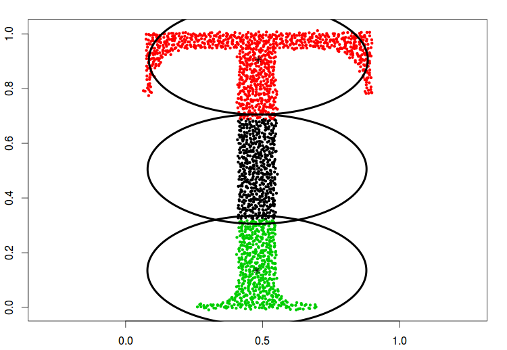In the above example, the following covariance matrix has been used, which results in covering the data by fixed shape ellipses: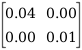### Fixed eigenvalues CEC

`type = "eigenvalues"`

Model based on Gaussians with arbitrary fixed eigenvalues.

``````data("Tset")

cec <- cec(x = Tset, centers = 10, type = "eigenvalues", param = c(0.01, 0.001), nstart = 5)

plot(cec, asp = 1)
``````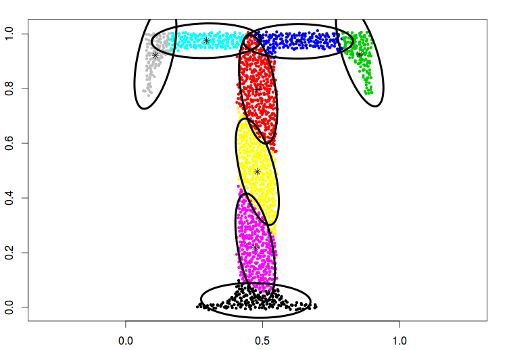In the above example, two eigenvalues: λ₁=0.01 and λ₂=0.001 are used, which results in covering the data with ellipses having fixed semi axes (corresponding to the eigenvalues).

### Fixed mean CEC

`type = "mean"`

Model based on Gaussians with fixed means.

``````data("Tset")
data("threeGaussians")

cec <- cec(Tset, 4, "mean", param = c(0.48, 0.48), nstart = 5)
plot(cec, asp = 1)

cec <- cec(threeGaussians,4, "mean", param = c(0, 0), nstart = 10)
plot(cec)
``````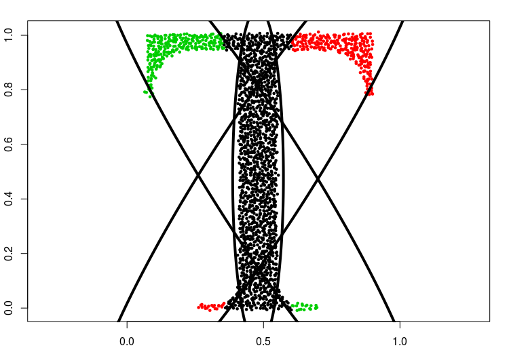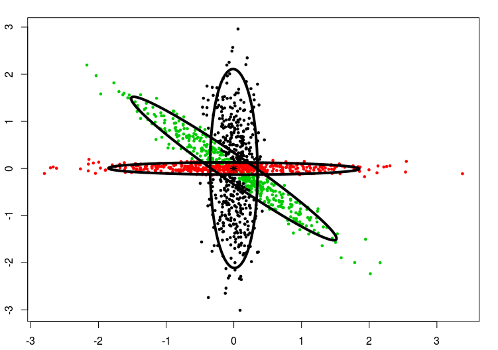## A mix of the Gaussian models

One of the most powerful properties of the CEC algorithm is the possibility of mixing models. More precisely, the mixed models can be specified by giving a list of cluster types (and a list of parameters if needed).

``````cec(x = ..., centers = ..., type = c("all", "diagonal", ...), param = ...).
``````
``````data("mixShapes")

cec <- cec(mixShapes, 7, iter.max = 3,
type = c("fixedr", "fixedr", "eigen", "eigen",  "eigen", "eigen", "eigen"),
param = list(350, 350, c(9000, 8), c(9000, 8), c(9000, 8), c(9000, 8), c(9000, 8)), nstart = 500)

plot(cec, asp = 1)
``````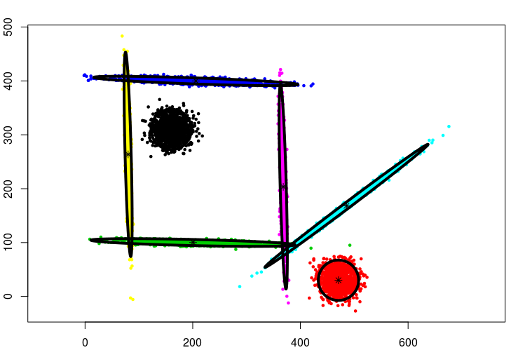## CEC Split

CEC Split method discovers new clusters after initial clustering, by recursively trying to split each cluster into two with lower cost function.

To enable split method, the `split` argument must be set to `TRUE`.

``````cec <- cec(x = ...,  centers = ..., split = T)
``````

There are four parameters (with their default values) that control the split mode: `split.depth = 8` , `split.tries = 5`, `split.limit = 100`, `split.initial.starts = 1`. The description of those parameters is provided in the package manual. Using `nstart` parameter, the whole procedure, from start to end (initial clustering and splitting), can be repeated multiple times. In this, case we can also use multiple threads (`threads` parameter).

An example usage is presented below:

``````data("fourGaussians")

cec <- cec(fourGaussians, centers = 1, type = "all", split = T)

plot(cec, asp = 1)
``````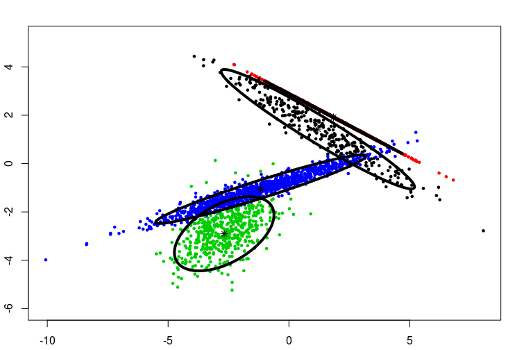The split method will be used implicitly when `centers` argument is not provided.

``````data("mixShapes")

cec <- cec(mixShapes)

plot(cec, asp = 1)
``````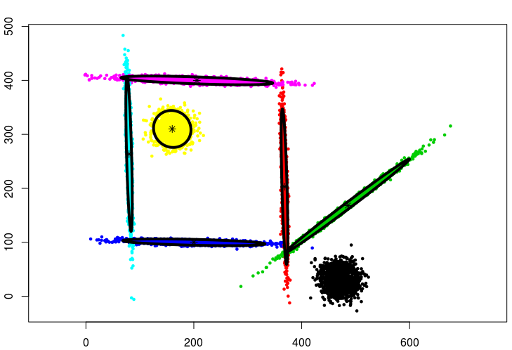The next two examples show clustering results using split method on a data set of 20 gaussians generated by the following code:

``````twenty.gaussians = matrix(c(0, 0), 0, 2)
for (i in 0:(19)) {
G = matrix(rnorm(400), 200, 2)
G[,1] = G[,1] + i %% 5 * 8 + runif(1,-1, 1)
G[,2] = G[,2] + i %/% 5 * 8 + runif(1,-1, 1)
twenty.gaussians = rbind(twenty.gaussians, G)
}
``````

In the following example, the usage of general gaussian distributions (`type = 'all'`) doesn't require any modification of default split parameters. Only the `card.min` needs to be set to a much lower value.

``````cec <- cec(twenty.gaussians, card.min="1%")

plot(cec, asp = 1)
``````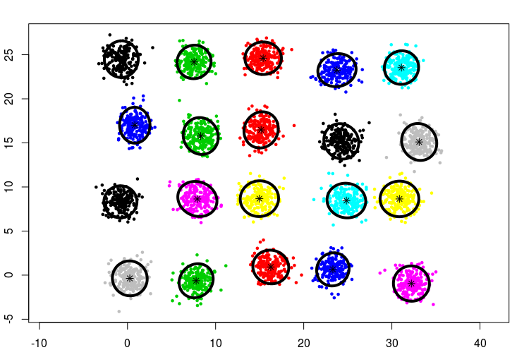Some data sets may require tuning of the split parameters. Using spherical densities (`type = 'spherical'`) on the same data, the `split.depth` needs to be increased significantly as well as `split.tries`. As in the previous example the `card.min` is changed.

``````cec <- cec(twenty.gaussians,, "sp", split.depth = 25, split.tries=15, card.min="1%")

plot(cec, asp = 1)
``````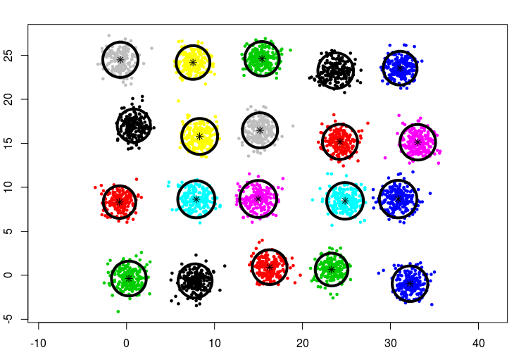## Try the CEC package in your browser

Any scripts or data that you put into this service are public.

CEC documentation built on Sept. 21, 2021, 5:07 p.m.